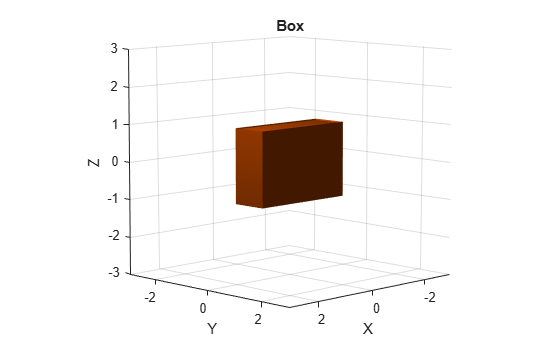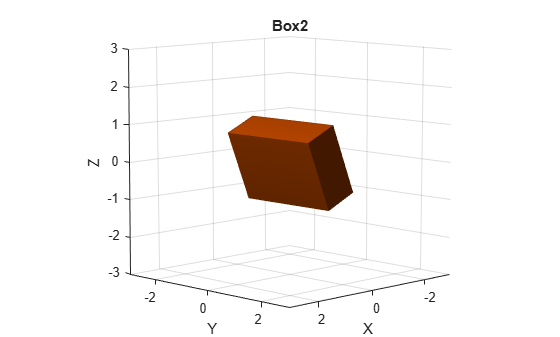# collisionBox

Create box collision geometry

Since R2019b

## Description

Use `collisionBox` to create a box collision geometry centered at the origin.

## Creation

### Syntax

``BOX = collisionBox(X,Y,Z)``
``BOX = collisionBox(___,Pose=pose)``

### Description

example

````BOX = collisionBox(X,Y,Z)` creates an axis-aligned box collision geometry centered at the origin with X, Y, and Z as its side lengths along the corresponding axes in the geometry-fixed frame. By default, the geometry-fixed frame collocates with the world frame.```
````BOX = collisionBox(___,Pose=pose)` sets the `Pose` property of the box to `pose`, relative to the world frame.```

## Properties

expand all

Side length of box geometry along the x-axis, specified as a positive scalar. Units are in meters.

Data Types: `double`

Side length of box geometry along the y-axis, specified as a positive scalar. Units are in meters.

Data Types: `double`

Side length of box geometry along the z-axis, specified as a positive scalar. Units are in meters.

Data Types: `double`

Pose of the collision geometry relative to the world frame, specified as a 4-by-4 homogeneous matrix or an `se3` object. You can change the pose after you create the collision geometry.

Note

Note that when the pose is specified as an `se3` object, the `Pose` property stores the pose as a numeric 4-by-4 matrix.

Data Types: `single` | `double`

## Object Functions

 `show` Show collision geometry `convertToCollisionMesh` Convert collision primitive geometry into collision mesh geometry `fitCollisionCapsule` Fit collision capsule around collision geometry

## Examples

collapse all

Create a box collision geometry centered at the origin. The side lengths in the x-, y-, and z-directions are 3, 1, and 2 meters, respectively.

`box = collisionBox(3,1,2)`
```box = collisionBox with properties: X: 3 Y: 1 Z: 2 Pose: [4x4 double] ```

Visualize the box.

```show(box) title("Box")```Create two homogeneous transformation matrices. The first matrix is a rotation about the z-axis by $\pi /2$ radians, and the second matrix is a rotation about the x-axis of $\pi /8$ radians.

```matZ = axang2tform([0 0 1 pi/2]); matX = axang2tform([1 0 0 pi/8]);```

Create a second box collision geometry with the same dimensions as the first. Change its pose to the product of the two matrices. The product corresponds to first rotation about the z-axis followed by rotation about the x-axis. Visualize the result.

```box2 = collisionBox(3,1,2); box2.Pose = matZ*matX; show(box2) title("Box2")```## Version History

Introduced in R2019b

expand all